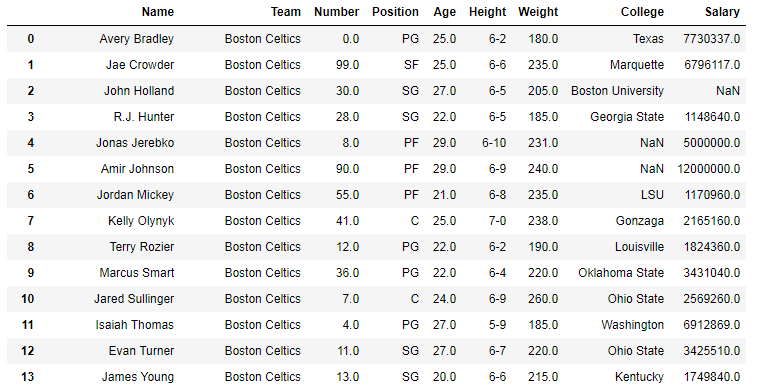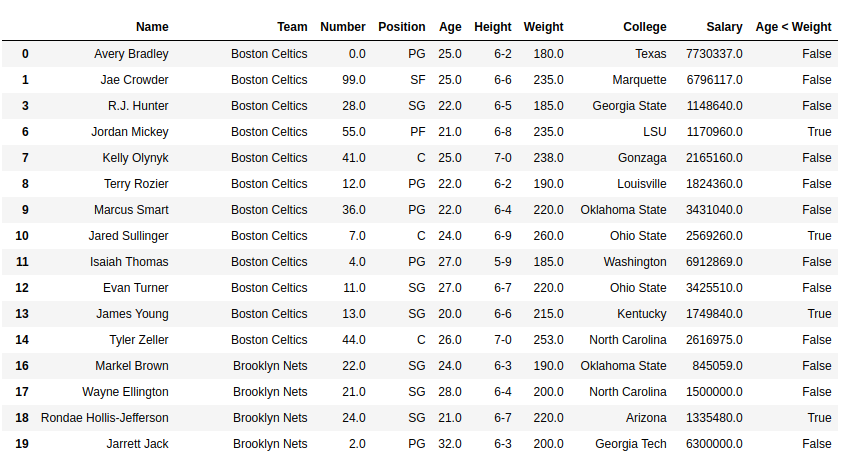# Python | Pandas Series.lt()

Python is a great language for doing data analysis, primarily because of the fantastic ecosystem of data-centric Python packages. Pandas is one of those packages and makes importing and analyzing data much easier.

Pandas `Series.lt()` is used to compare two series and return Boolean value for every respective element.

Syntax: Series.lt(other, level=None, fill_value=None, axis=0)

Parameters:
other: other series to be compared with
level: int or name of level in case of multi level
fill_value: Value to be replaced instead of NaN
axis: 0 or ‘index’ to apply method by rows and 1 or ‘columns’ to apply by columns.

Return type: Boolean series

Note: The results are returned on the basis of comparison caller series < other series.

In the following examples, the data frame used contains data of some NBA players. The image of data frame before any operations is attached below.Example #1:

In this example, the Age column and Weight columns are compared using .lt() method. Since values in weight columns are very large as compared to Age column, hence the values are divided by 10 first. Before comparing, Null rows are removed using .dropna() method to avoid errors.

 `# importing pandas module  ` `import` `pandas as pd  ` ` `  `# making data frame  ` `data ``=` `pd.read_csv(``"https://media.geeksforgeeks.org/wp-content/uploads/nba.csv"``)  ` `   `  `# removing null values to avoid errors  ` `data.dropna(inplace ``=` `True``)  ` ` `  `# other series ` `other ``=` `data[``"Weight"``]``/``10` ` `  `# calling method and returning to new column ` `data[``"Age < Weight"``]``=` `data[``"Age"``].lt(other) ` ` `  `# display ` `data `

Output:
As shown in the output image, the new column has True wherever value in Age column is less than Weight/10.Example #2: Handling NaN values

In this example, two series are created using `pd.Series()`. The series contains null value too and hence 10 is passed to fill_value parameter to replace null values by 10.

 `# importing pandas module  ` `import` `pandas as pd  ` ` `  `# importing numpy module ` `import` `numpy as np ` ` `  `# creating series 1 ` `series1 ``=` `pd.Series([``11``, ``21``, ``2``, ``43``, ``9``, ``27``, np.nan, ``110``, np.nan]) ` ` `  `# creating series 2 ` `series2 ``=` `pd.Series([``16``, np.nan, ``2``, ``23``, ``5``, ``40``, np.nan, ``0``, ``19``]) ` ` `  `# setting null replacement value ` `na_replace ``=` `10` ` `  `# calling and storing result ` `result ``=` `series1.lt(series2, fill_value ``=` `na_replace) ` ` `  `# display ` `result `

Output:
As it can be seen in output, NaN values were replaced by 5 and the comparison is performed after the replacement and new values are used for comparison.

```0     True
1    False
2    False
3    False
4    False
5     True
6    False
7    False
8     True
dtype: bool
```

My Personal Notes arrow_drop_upCheck out this Author's contributed articles.

If you like GeeksforGeeks and would like to contribute, you can also write an article using contribute.geeksforgeeks.org or mail your article to contribute@geeksforgeeks.org. See your article appearing on the GeeksforGeeks main page and help other Geeks.

Please Improve this article if you find anything incorrect by clicking on the "Improve Article" button below.

Improved By : Akanksha_Rai

Article Tags :

1

Please write to us at contribute@geeksforgeeks.org to report any issue with the above content.